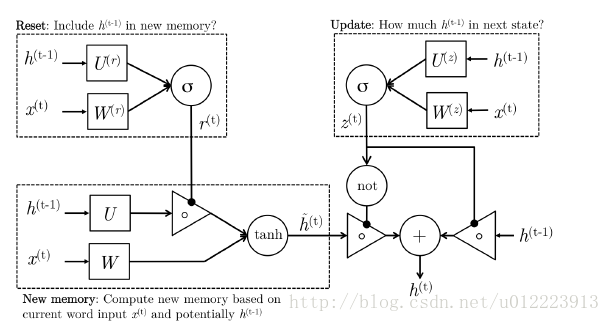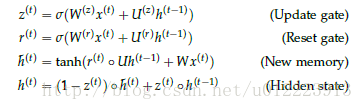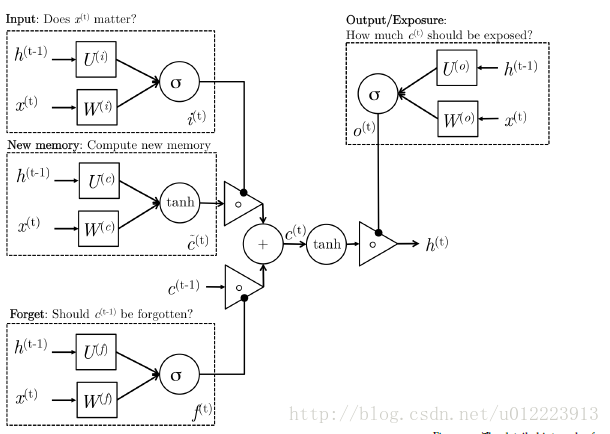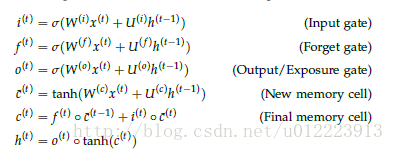# The difference between LSTM and Gru

First, some conclusions are given

The performance of Gru and LSTM is equal in many tasks. With fewer Gru parameters, it is easier to converge, but LSTM performs better when the data set is large. Structurally, Gru has only two gates (update and reset), LSTM has three gates (forge, input and output). Gru directly transfers the hidden state to the next unit, while LSTM packages the hidden state with memory cell.

1. Basic structure

1.1 GRUGru is designed to better capture long-term dependencies. Let’s look at the input first

ht−1

and

x(t)

How can Gru calculate the output

h(t)Reset gate

r(t)

Responsible for decision making

h(t−1)

For new memory

h^(t)

How important is it if

r(t)

If it’s about 0,

h(t−1)

It will not be passed to new memory

h^(t)

new memory

h^(t)

It’s a new input

x(t)

And the hidden state of the previous moment

h(t−1)

The summary of this paper. Calculate the new vector summed up

h^(t)

Contains the above information and new input

x(t)

. Update gate

z(t)

Responsible for deciding how much to pass

ht−1

to

ht

. If

z(t)

If it’s about one,

ht−1

Almost directly copied to

ht

On the contrary, if

z(t)

About 0, new memory

h^(t)

Pass directly to

ht

. Hidden state:

h(t)

from

h(t−1)

and

h^(t)

The weight of the two is determined by update gate

z(t)

Control. ​

1.2 LSTMLSTM is also designed to better capture long-term dependencies, but the structure is different and more complex. Let’s take a look at the calculation process:The new memory cell step is similar to the new memory in Gru. The output vector

c^(t)

It’s all about new input

x(t)

And the hidden state of the previous moment

h(t−1)

The summary of this paper. Input gate

i(t)

Responsible for determining input

x(t)

Whether the information is worth saving. Forget gate

f(t)

Responsible for determining past memory cell

c^(t−1)

yes

c(t)

It’s important. final memory cell

c(t)

from

c^(t−1)

and

c^(t)

The weight is determined by forge gate and input gate respectively. The output gate is not available in Gru. It’s responsible for making decisions

c(t)

Which parts of should be passed to hidden state

h(t)

2. Difference

1. Control of memory

LSTM: controlled by output gate and transmitted to the next unit

Gru: pass it directly to the next unit without any control

2. Input gate and reset gate have different action positions

LSTM: computing new memory

c^(t)

Instead of controlling the information of the previous moment, we use forge gate to achieve this independently

Gru: computing new memory

h^(t)

Reset gate is used to control the information of the previous time.

3. Similar

The biggest similarity is that addition is introduced in the update from t to T-1.

The advantage of this addition is that it can prevent gradient dispersion, so LSTM and Gru are better than RNN.

Reference：
1. https://cs224d.stanford.edu/lecture_ notes/LectureNotes4.pdf
2. Empirical Evaluation of Gated Recurrent Neural Networks on Sequence Modeling
3. https://feature.engineering/difference-between-lstm-and-gru-for-rnns/